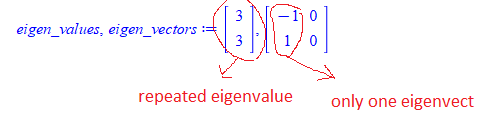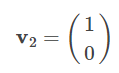# Question:Finding all Eigenvectors for real and repeated eigenvalue in Maple

## Question:Finding all Eigenvectors for real and repeated eigenvalue in Maple

Maple 2020

When a real matrix have repeated eigenvalue (i.e. multiplicity >1) and the matrix is not the identity matrix, then it is called defective eigenvalue.

For example if the eigenvalue is repeated 2 times, there will be only one eigevector associated with it.

There is an algorithm to generate another (linearly independent) eigenvector from this same eigenvalue called the defective eigenvalue method.

My question is: Does Maple have support for finding such additional eigenvectors?  The standard Eigenvectors does not seem to do this. Here is an example.

```restart;
A :=Matrix([[1,-2],[2,5]]);
(eigen_values, eigen_vectors) := LinearAlgebra:-Eigenvectors(A);
```By hand, using the defective eigenvalue method it is possible find second eigenvector for this eigenvalue, which is linearly independent of the one returned by Maple. Such asThe method to find these additional eigenvector is described in number of places such as

https://en.wikipedia.org/wiki/Defective_matrix

And the pdf at the bottom show a more detailed example.

I can code this method by hand to find complete set of L.I. eigenvectors for this defective eigenvalue.

But before doing that, I thought to ask if Maple have buildin support for this, such as a single command to do this or some package.

I looked at help for LinearAlgebra and I am still not able to see anything, It only says this:

With an eigenvalue of multiplicity  k>1 , there may be fewer than  k linearly independent eigenvectors. In this case, the matrix is called defective.  By design, the returned matrix always has full column dimension.  Therefore, in the defective case, some of the columns that are returned are zero.  Thus, they are not eigenvectors.  With the option, output=list, only eigenvectors are returned.  For more information, see LinearAlgebra[JordanForm] and LinearAlgebra[SchurForm].

This PDF also describes using Maple, how to do it for one example

https://wps.prenhall.com/wps/media/objects/884/905485/chapt5/proj5.4/proj5-4.pdf

This PDF also describes how to do it by hand without using Maple, starting at end of page 3

http://www.math.utah.edu/~zwick/Classes/Fall2013_2280/Lectures/Lecture23_with_Examples.pdf

It is not too hard to code this method in Maple. But if Maple allready can do it, using some command, it will be better ofcourse.﻿